Courses

# Design Of Band And Disc Brakes Mechanical Engineering Notes | EduRev

## Mechanical Engineering : Design Of Band And Disc Brakes Mechanical Engineering Notes | EduRev

The document Design Of Band And Disc Brakes Mechanical Engineering Notes | EduRev is a part of the Mechanical Engineering Course Machine Design.
All you need of Mechanical Engineering at this link: Mechanical Engineering

Band brakes:

The operating principle of this type of brake is the following. A flexible band of leather or rope or steel with friction lining is wound round a drum. Frictional torque is generated when tension is applied to the band. It is known (see any text book on engineering mechanics) that the tensions in the two ends of the band are unequal because of friction and bear the following relationship: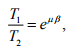where T1= tension in the taut side,
T2 = tension in the slack side,
μ = coefficient of kinetic friction and
β = angle of wrap.

If the band is wound around a drum of radius R, then the braking torque is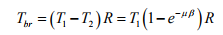Depending upon the connection of the band to the lever arm, the member responsible for application of the tensions, the band brakes are of two types,

(a) Simple band brake:

In simple band brake one end of the band is attached to the fulcrum of the lever arm (see figures 12.2.1(a) and 1(b) ). The required force to be applied to the lever is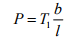for clockwise rotation of the brake drum and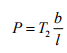for anticlockwise rotation of the brake drum,

where l = length of the lever arm and
b = perpendicular distance from the fulcrum to the point of attachment of other end of the band.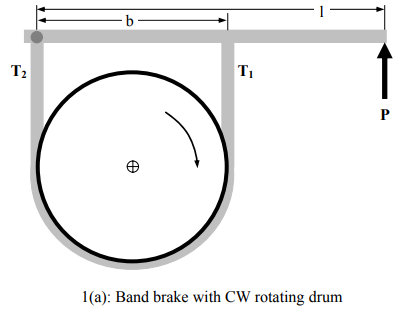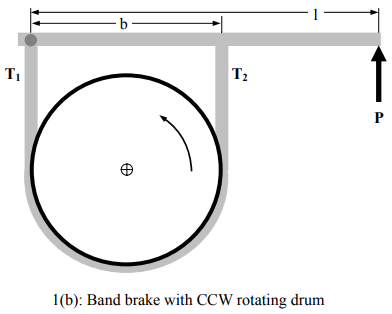Figure 12.2.1: Band brakes

(b) Differential band brake:

In this type of band brake, two ends of the band are attached to two points on the lever arm other than fulcrum (see figures 12.2.2(a) and 12.2.2(b)). Drawing the free body diagram of the lever arm and taking moment about the fulcrum it is found that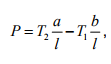for clockwise rotation of the brake drum and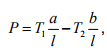foranti clockwise rotation of the brake drum and

Hence, P is negative if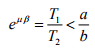for clockwise rotation of the brake drum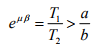for counterclockwise rotation of the brake drum. In

these cases the force is to be applied on the lever arm in opposite direction to maintain equilibrium. The brakes are then self locking.

The important design variables of a band brake are the thickness and width of the band. Since the band is likely to fail in tension, the following relationship is to be satisfied for safe operation.

T1 = wtsT

where w = width of the band,
t = thickness of the band and
sT= allowable tensile stress of the band material. The steel bands of the following dimensions are normally used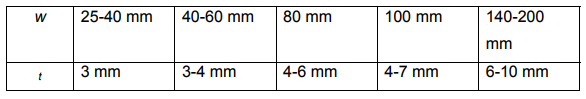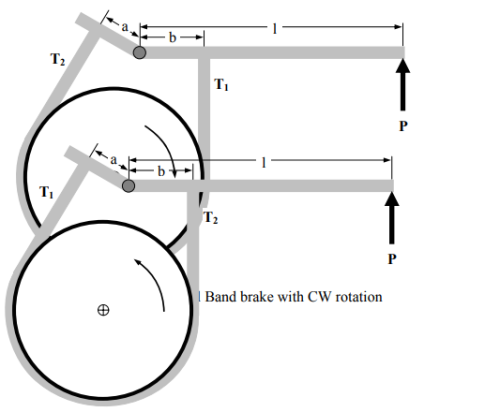Fig 12.2.2(b): Differential Band brake with CCW rotation

Band and block brakes:

Sometimes instead of applying continuous friction lining along the band, blocks of wood or other frictional materials are inserted between the band and the drum. In this case the tensions within the band at both sides of a block bear the relation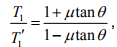whereT1 = tension at the taut side of any block
T ′1 = tension at the slack side of the same block
2θ = angle subtended by each block at center.
If n number of blocks are used then the ratio between the tensions at taut side to slack side becomes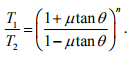The braking torque is Tbr =  (T1- T2 )  R

Disc brake:

In this type of brake two friction pads are pressed axially against a rotating disc to dissipate kinetic energy. The working principle is very similar to friction clutch. When the pads are new the pressure distribution at pad-disc interface is uniform, i.e.

p = constant .

If F is the total axial force applied then p =  F/A, where A is the area of the pad. The frictional torque is given by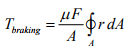where μ = coefficient of kinetic friction and r is the radial distance of an infinitesimal element of pad. After some time the pad gradually wears away. The wear becomes uniforms after sufficiently long time, when

pr = constant = (say)

where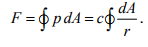The braking torque is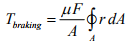It is clear that the total braking torque depends on the geometry of the pad. If the annular pad is used then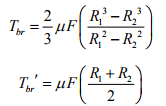where R1 and R2 are the inner and outer radius of the pad.

Friction materials and their properties.

The most important member in a mechanical brake is the friction material. A good friction material is required to possess the following properties:

• High and reproducible coefficient of friction.
• Imperviousness to environmental conditions.
• Ability to withstand high temperature (thermal stability)
• High wear resistance.
• Flexibility and conformability to any surface.

Some common friction materials are woven cotton lining, woven asbestos lining, molded asbestos lining, molded asbestos pad, Sintered metal pads etc.

Offer running on EduRev: Apply code STAYHOME200 to get INR 200 off on our premium plan EduRev Infinity!

## Machine Design

57 videos|71 docs|102 tests

,

,

,

,

,

,

,

,

,

,

,

,

,

,

,

,

,

,

,

,

,

;